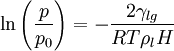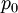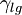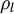# Kelvin equation$\ln \left( \frac{p}{p_0}\right) = - \frac{2\gamma_{lg}}{RT\rho_lH}$
Where p is the pressure,$p_0$ is the saturated vapour pressure,$\gamma_{lg}$ is the liquid-gas surface tension for the bulk fluid,$\rho_l$ is the density of the bulk fluid, R is the molar gas constant, T is the temperature and H is the pore or capillary width.